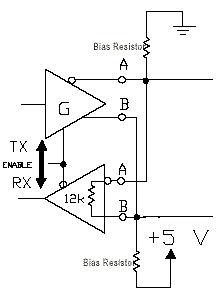# Remote Device Management (RDM)

## RDM Physical Layer

Two important additional physical layer requirements for RDM are that the controller output must be terminated and it must provide a bias circuit to keep the cable in the ‘marking state’ when no driver is enabled. The first requirement is needed because other devices may send signals on the DMX bus and hence it is essential that bot ends of the cable are terminated (it was good practice anyway with standard DMX). The second requirement arises because the controller does not always send a signal - this means that the conductors would float and could easily pickup noise, instead RDM requires the controller to use a bias network.

## RDM Bias Network

During RDM transactions there are short times when the controller has requested data from an RDM device and has changed into receive-mode waiting for a reply. Until the device takes control of the line to send a reply the line is in an unconnected state with no transmitter to hold the two wires in the idle condition. Unless the line is externally forced to the idle condition it could be prone to noise that could be misinterpreted by receivers as the start bit of a data byte.The RDM bias network using two bias resistors, each of 510 Ohms.

If there is no sender the two data lines "float", which could allow received noise to be larger than the detection threshold.

The RDM spec says "The command port shall provide a means to bias the termination of the data link to a value of at least 245 mV". This is achieved using an RDM line biasing network. The bias circuit prevents noise from being seen as a signal. Bias is provided by a pair of resistors arranged to hold the data lines at a known state (generating a difference between the data lines of at least 245 mV) when no transmitter is driving the DMX line.

One, and one only, of the connected devices should use an RDM line biasing network.

##### Calculation of bias resistance

Calculation of the bias resistance, R.

The conductor A is connected via the bias resistance R to GND
The conductor B is connected via the bias resistance R to +5V

Each DMX receiver has an input impedance of 12K.
32 nodes in parallel therefore present a combined load of 376 ohms.

Total load is therefore 1/(0.0167+0.0026)=51.8 Ohms

To maintain at least 245 mV across the balanced pair therefore using Ohm's law, the required current through the bias network is ~ 4.7 mA to flow through this load.

To create this bias from a 5V supply will need a total series resistance of 1063 Ohms. Some of this load will be supplied by the termination/cable impedance Therefore, subtract 51.8 Ohms contributed by the line/terminators, this leaves 1011 Ohms to be provided as series bias.

Placing half this bias resistance as a pull-up to 5V and half as a pull-down to ground gives a bias R of 505 Ohms. 510 Ohms is the nearest preferred value.